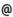## Babes-Bolyai University of Cluj-Napoca Faculty of Mathematics and Computer Science Study Cycle: Master SUBJECT

 Code Subject MMA1015 Univalent Functions
 Section Semester Hours: C+S+L Category Type Mathematics Computational - in Hungarian 4 2+2+0 speciality optional Didactic Mathematics - in Hungarian 4 2+2+0 speciality optional
 Teaching Staff in Charge
 Prof. BULBOACA Teodor, Ph.D.,  bulboacamath.ubbcluj.ro
 Aims The presentation of principal classes of univalent functions defined by remarkable geometric properties and some of their applications in the theory of conformal mappings.
 Content 1. Univalent functions; classical results. (6 hours lectures + 6 hours seminar) - Aria@s Theorem. Covering Theorem for the S class (Koebe, Bieberbach). Covering Theorem for the Sigma class. - Distortion Theorems (Koebe, Bieberbach). The compactness of the S class. The Bieberbach@s conjecture. 2. Analytical functions with real positive part. Subordination. (6 hours lectures + 4 hours seminar + 2 hours test) - Integral representation; Herglotz@s formula. The theorems of Herglotz. - Representations by Stiltjes integrals. Caratheodory@s Theorem. - Bounds of holomorphic functions with real positive part. - Subordination; the subordination principle (Lindelof). Sakaguchi@s Lemma. 3. Special classes of univalent functions. (12 hours lectures + 12 hours seminar) - Starlike functions. Radius of starlikeness. Theorem about the coefficient bounds of functions from S^*. Structure formula. - Convex functions. Duality@s Theorem (Alexander). The compactness of K class. Radius of convexity. - Alpha - convex functions. The Theorem of starlikeness of alpha - convex functions. Duality@s Theorem. Radius of alpha - convexity. Bounds Theorems (Miller). - Close - to - convex functions. Univalence criteria of Noshiro - Warschawski - Wolff. Univalence criteria of Ozaki - Kaplan. Characterizing Theorem of close - to - convex functions (Kaplan). Linear accessible domains. - Typical real functions. Structure formula. Duality Theorem. Theorem about the coefficients. A sufficient condition for the univalence of the typical real functions. Consequence (Aksentiev). Thalk - Chakalov Theorem. Univalence criteria for meromorphic functions. Aksentiev@s Theorem. Starlikeness and convexity conditions for meromorphic functions. 4. Diffeomorphism conditions in the complex plane. (4 hours lectures + 2 hours seminar + 2 hours test) - Spirallike generalized functions of C^1 class. General theorems; particular cases. - Nonanalytic alpha - convex function. Preliminary lemmas. The Theorem of starlikeness of alpha - convex nonanalytic functions. Examples. - C^1 transforms and the refraction law.
 References - Bibliografia obligatorie: 1. Bulboacă, Teodor - Mocanu, Petru : Bevezetés az analitikus függvények geometriai elméletébe, Editura Abel, Cluj-Napoca, 2003. 2. Mocanu, Petru - Bulboacă, Teodor - Sălăgean, Gr. Ştefan : Teoria geometrică a funcţiilor univalente, ediţia a II-a, Editura Casa Cărţii de Ştiinţă, Cluj-Napoca, 2006. - Bibliografia opţională: Următoarele cărţi pot fi găsite la Biblioteca Facultăţii de Matematică şi Informatică: 1. Bulboacă, Teodor : Differential subordinations and superordinations. New Results, Editura Casa Cărţii de Ştiinţă, Cluj-Napoca, 2005. 2. Goluzin, G. M. : Geometric theory of functions of a complex variable, Trans. Math. Mon., Amer. Math. Soc., 1969. 3. Goodman, A. W. : Univalent functions (vol. I, II), Mariner Publishing Co., Tampa, 1983. 4. Duren, P. L. : Univalent functions, Springer Verlag, Berlin, Heidelberg, 1984. 5. Mocanu, Petru - Miller, S. Sanford : Differential Subordinations. Theory and Applications, M. Dekker, 2000.
 Assessment Exam. Student tests during the semester; their average represents 1/3 from the final score.
 Links: Syllabus for all subjects Romanian version for this subject Rtf format for this subject